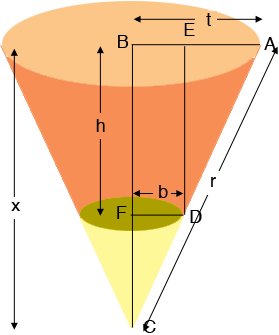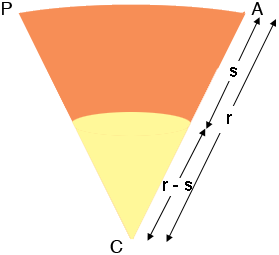SEARCH HOMEMath Central Quandaries & QueriesQuestion from Josh, a student: I need to know how to layout a truncated cone with a base diameter of 18" a top diameter 15.25 and a height of 20". Your help will be greatly appreciated.Hi Josh,

Here is my diagram of your truncated cone, the diagram is certainly not to scale. I also drew it upside down to the way you described it.I extended the sides of the truncated cone to meet at $C$ and form a full cone. In your situation the diameter at the top is $D = 18"$ and the diameter at the bottom $d = 15.25"$ and $h = 20".$ Thus $t = 9"$ and $b = 7.625".$ Triangles $ABC$ and $DFC$ are similar so

$\frac{x}{t} = \frac{h}{t - b}$

and hence

$x = \frac{ht}{t - b}.$

The slant height $|CA| = r$ is given by Pythagoras Theorem as

$r = \sqrt{\left( \frac{ht}{t - b} \right)^2 + t^2}$

Similarly the slant height $|DA|$ of the truncated cone is given by

$s = |DA| = \sqrt{(t - b)^2 + h^2}.$

Now slice the cone from $A$ to $C$ and lie it flat.The length of the arc from $A$ to $P$ is the circumference of the large end of the truncated cone so the length of the arc from $A$ to $P$ is $2 \pi \; t$ units. The angle $ACP$ is a fraction of the $360^o$ that makes up the circle. That fraction is

$\frac{2 \pi \; t}{2 \pi \; r} \times 360^o = \frac{t}{r} \times 360^o$

The construction is then to draw two circles with radii $r$ units, in your case inches, and the other with radius $r - s$ units, each with the same center P. Cut out the wedge $ACP$ with center angle measure of $\frac{t}{r} \times 360$ degrees. Roll up the wedge to form the truncated cone. I don't know what material you are using but I would do it on scrap material first.

Penny

In March of 2014 Don Nevin, a retired mathematician from Vermont Technical College, sent us an Excel spreadsheet which can be used to calculate the dimensions required to produce a flat template for a truncated cone. Don kindly gave us permission to make his spreadsheet available to Math Central users. You can download his spreadsheet here. Notice that in Penny's response above the wedge $ACP$ is the piece to be rolled up to form the truncated cone. In Don's spreadsheet the angle $\Theta$ is the central angle of the sector to be removed so that the remainder is to be rolled up to form truncated cone. Thus $\frac{t}{r} \times 360 = 360 - \Theta.$

Thanks Don.Math Central is supported by the University of Regina and The Pacific Institute for the Mathematical Sciences.